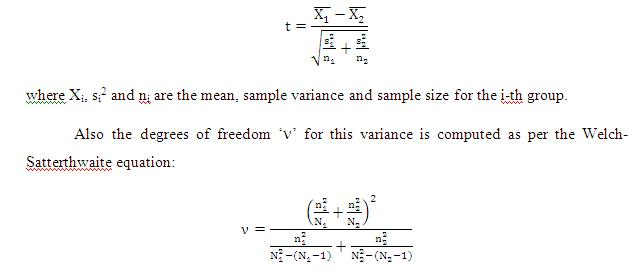# Welch-Satterthwaite T-Test - Meaning & Definition

## What is Welch-Satterthwaite T-Test?

In case where one has to compare two non-paired groups with unequal variances, the standard t-test which assumes equality if variances of the two groups, cannot be used. The test then used is called the Welch-Satterthwaite t-test.

Method:

The null hypothesis in case of two groups with unequal variances is that the two variances are different. Such a situation may arise while comparing two production processes for comparing line performance. This assumption has to be affirmed or negated through the test.

In the normal Student’s t-test, in case of two groups, as the variances are assumed to be pooled and equal, the t-statistic (parameter to be tested for a hypothesis) is simply the difference in two means divided by the single standard error (variance divided by sample size). But in this case, as the variances differ by the problem definition, the t-statistic is computed differently as:Then the standard procedure is followed by comparing the t-statistic with the t-distribution table. If the value of the t-statistic lies outside as specified by the confidence interval in question, then the null hypothesis is rejected, otherwise it cannot be rejected.

This article has been researched & authored by the Business Concepts Team. It has been reviewed & published by the MBA Skool Team. The content on MBA Skool has been created for educational & academic purpose only.

Browse the definition and meaning of more similar terms. The Management Dictionary covers over 2000 business concepts from 5 categories.